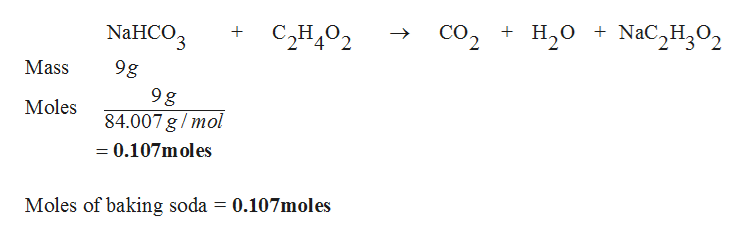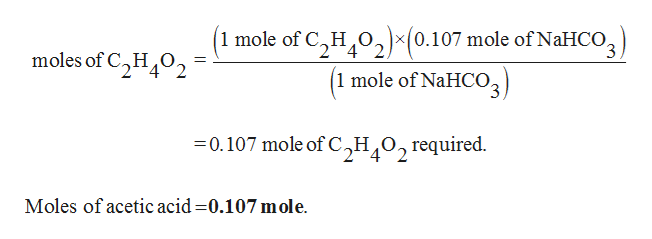# Baking soda and vinegar react according to the following chemical equation: NaHCO3+C2H4O2→CO2+H2O+NaC2H3O2 Determine how much vinegar would be needed to react with 9 grams (approximately 1 tbsp.) of baking soda by answering the following questions.How many moles of molecules of baking soda are in 9 grams of baking soda? How many moles of acetic acid are needed to fully react with the number of moles of baking soda in the recipe? Commercial distilled vinegar is approximately 1 M acetic acid. How many mL of distilled vinegar are needed to fully react with the baking soda?

Question
16 views

Baking soda and vinegar react according to the following chemical equation:

NaHCO3+C2H4O2→CO2+H2O+NaC2H3O2

Determine how much vinegar would be needed to react with 9 grams (approximately 1 tbsp.) of baking soda by answering the following questions.

How many moles of molecules of baking soda are in 9 grams of baking soda?

How many moles of acetic acid are needed to fully react with the number of moles of baking soda in the recipe?

Commercial distilled vinegar is approximately 1 M acetic acid. How many mL of distilled vinegar are needed to fully react with the baking soda?

check_circle

star
star
star
star
star
1 Rating
Step 1

Moles of baking soda is calculated for the given reaction as follows,help_outlineImage Transcriptioncloseсо, + н,о + NaC,H,0, + Сно, 2 NaHCO3 9g Mass 9 g 84.007 g /mol Moles =0.107moles Moles of baking soda = 0.107moles fullscreen
Step 2

Hence, 1 mole of NaHCO3 requires 1 mole of C2H4O2, then

0.107 moles of NaHCO3 requires ‘x&...help_outlineImage Transcriptionclose(1 mole of C2H202 (0.107 mole of NaHCo3 moles of C2H 2 (1 mole of NaHCO2 0.107 mole of C,H O, required Moles of acetic acid 0.107 mole fullscreen

### Want to see the full answer?

See Solution

#### Want to see this answer and more?

Solutions are written by subject experts who are available 24/7. Questions are typically answered within 1 hour.*

See Solution
*Response times may vary by subject and question.
Tagged in

### Chemistry